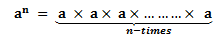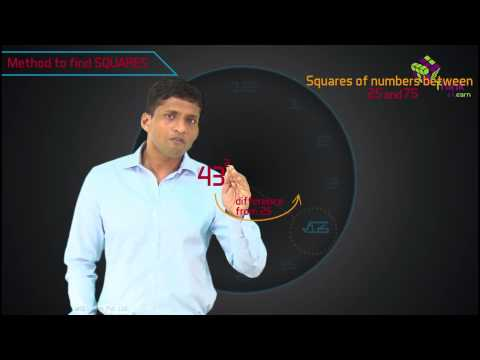# Laws Of Exponents

In Mathematics, there are different laws of exponents. All the rules of exponents are used to solve many mathematical problems which involve repeated multiplication processes. The laws of exponents simplify the multiplication and division operations and help to solve the problems easily. In this article, we are going to discuss the six important laws of exponents with many solved examples.

## What are Exponents?

Exponents are used to show repeated multiplication of a number by itself. For example, 7 × 7 × 7 can be represented as 73. Here, the exponent is ‘3’ which stands for the number of times the number 7 is multiplied. 7 is the base here which is the actual number that is getting multiplied. So basically exponents or powers denotes the number of times a number can be multiplied. If the power is 2, that means the base number is multiplied two times with itself. Some of the examples are:

• 34 = 3×3×3×3
• 105 = 10×10×10×10×10
• 163 = 16 × 16 × 16

Suppose, a number ‘a’ is multiplied by itself n-times, then it is represented as an where a is the base and n is the exponent.Exponents follow certain rules that help in simplifying expressions which are also called its laws. Let us discuss the laws of exponents in detail.

## Rules of Exponents With Examples

As discussed earlier, there are different  laws or rules defined for exponents. The important laws of exponents are given below:

• am×an = am+n
• am/an = am-n
• (am)n = amn
• an/bn = (a/b)n
• a0 = 1
• a-m = 1/am
• $$\begin{array}{l}a^{\frac{1}{n}}=\sqrt[n]{a}\end{array}$$

Also, read: Now let us discuss all the laws one by one with examples here.

### Product With the Same Bases

As per this law, for any non-zero term a,

• am×a= am+n

where m and n are real numbers.

Example 1: What is the simplification of 55 × 51 ?

Solution: 55 × 51 = 55+1 = 56

Example 2: What is the simplification of (−6)-4 × (−6)-7?

Solution: (−6)-4 × (−6)-7 = (-6)-4-7 = (-6)-11

Note: We can state that the law is applicable for negative terms also. Therefore the term m and n can be any integer.

### Quotient with Same Bases

As per this rule,

• am/an = am-n

where a is a non-zero term and m and n are integers.

Example 3: Find the value when 10-5 is divided by 10-3.

Solution: As per the question;

10-5/10-3

= 10-5-(-)3

= 10-5+3

= 10-2

= 1/100

### Power Raised to a Power

According to this law, if ‘a’ is the base, then the power raised to the power of base ‘a’ gives the product of the powers raised to the base ‘a’, such as;

• (am)n = amn

where a is a non-zero term and m and n are integers.

Example 4: Express 83 as a power with base 2.

Solution: We have, 2×2×2 = 8 = 23

Therefore, 83= (23)3 = 29

### Product to a Power

As per this rule, for two or more different bases, if the power is same, then;

• ab= (ab)n

where a is a non-zero term and n is the integer.

Example 5: Simplify and write the exponential form of: 1/8 x 5-3

Solution: We can write, 1/8 = 2-3

Therefore, 2-3 x 5-3 = (2 × 5)-3 = 10-3

### Quotient to a Power

As per this law, the fraction of two different bases with the same power is represented as;

•  an/b= (a/b)n

where a and b are non-zero terms and n is an integer.

Example 6: Simplify the expression and find the value:153/53

Solution: We can write the given expression as;

(15/5)3= 33 = 27

### Zero Power

According to this rule, when the power of any integer is zero, then its value is equal to 1, such as;

a= 1

where ‘a’ is any non-zero term.

Example 7: What is the value of 50 + 22 + 40 + 71 – 31 ?

Solution: 50 + 22 + 40 + 71 – 31 = 1+4+1+7-3= 10

### Negative Exponent Rule

According to this rule, if the exponent is negative, we can change the exponent into positive by writing the same value in the denominator and the numerator holds the value 1.

The negative exponent rule is given as:

a-m = 1/am

Example 8:

Find the value of 2-2

Solution:

Here, the exponent is a negative value (i.e., -2)

Thus, 2-2 can be written as 1/22

2-2 = 1/22

2-2 = 1/4

In other words, we can say that, if “a” is a non-zero number or non-zero rational number, we can say that a-m is the reciprocal of am.

### Fractional Exponent Rule

The fractional exponent rule is used, if the exponent is in the fractional form. The fractional exponent rule is given by:

$$\begin{array}{l}a^{\frac{1}{n}}=\sqrt[n]{a}\end{array}$$

Here, a is called the base, and 1/n is the exponent, which is in the fractional form. Thus, a1/n is said to be the nth root of a.

Example 9:

Simplify: 41/2

Solution:

Here, the exponent is in fractional form. (i.e., ½)

According to the fractional exponent rule, 41/2 can be written as √4

(i.e.,) 41/2 = √4

41/2 = 2 (As, the square root of 4 is 2)

Hence, the simplified form of 41/2 is 2.### Practice Problems on Laws of Exponents

Simplify the following expressions using the laws of exponents:

1. (42)3
2. 42×47
3. 3-3
4. 641/2
5. 70×23

## Frequently Asked Questions on Laws of Exponents

### What are exponents?

The exponents, also called powers, define how many times we have to multiply the base number. For example, the number 2 has to be multiplied 3 times and is represented by 23.

### What are the different laws of exponents?

The different Laws of exponents are:

• am×an = am+n
• am/an = am-n
• (am)n = amn
• an/bn = (a/b)n
• a0 = 1
• a-m = 1/am

### What is Power of a power rule?

In the power of a power rule, we have to multiply the exponent values. For example, (23)2 can be written as 26.

### Explain the zero power rule.

According to the zero power rule, if the exponent is zero, the result is 1, whatever the base value is. It means that anything raised to the power of 0 is 1. For example, 50 is 1.

### Simplify the expression 22.25

In expression 22.25, the base values are the same, So we can add the exponents.
Hence, 22.25 = 22+5
22.25 = 27.

Stay tuned with BYJU’S – The Learning App and download the app to get all the Maths concepts and learn in an easy way.

Test your knowledge on Laws Of Exponents